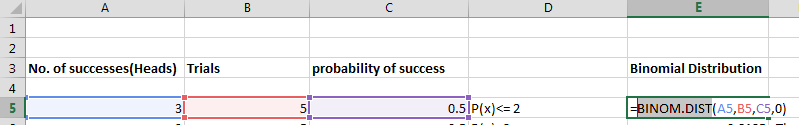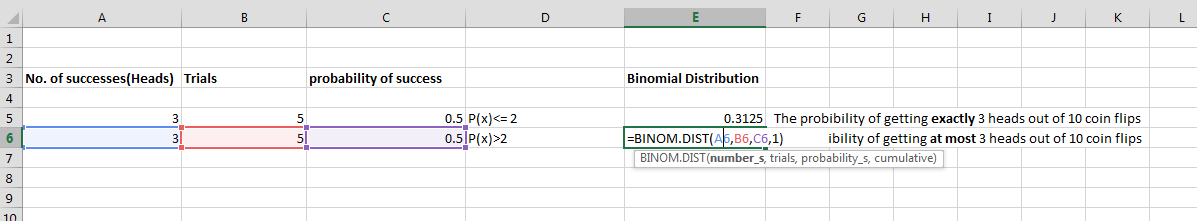## Binomial distribution in Excel

Excel has got many features connected with statistics. For examples Excel could help you to calculate binomial distribution (aka bernoulli distribution-“The Bernoulli distribution is a special case of the binomial distribution where a single trial is conducted (so n would be 1 for such a binomial distribution”). Let’s teach yourself how to do it in this easy steps.

## What is Binomial Distribution?

The binomial distribution is useful for describing a binomial (“zero-one”) process, for example, the number of women and men in a random sample from several companies or the number of defective items in a sample of 20 taken in a manufacturing process. The definition function is defined as: f(x) = [n!/ (x! * (n-x)!)] * px * qn-x, for x = 0,1,2, …, n

## Business example of Binominal Distribution

Here’s the real business example how you can use the binomial distribution in Excel.

The production of a your company products includes 35% of the 1st grade products, the rest are 2nd grade products. The customer purchased 10,000 items of products. Using binomial distribution calculate:

• the probability that only 3000 of the purchased products will be 1st grade
• the probability that only 3000 of the purchased products will be 2nd grade
• what average number of 1st grade products can the customer expect if he purchases 50,000 items

## COMBIN function

The first function in Excel to deal with the binomial distribution is COMBIN. This function calculates the binomial coefficient C (n, k), also known as the number of combinations of k elements from the set n. The two arguments of this function are the number of n trials and the k number of successes. Excel defines the function as follows:

=COMBIN (number, number_chosen)

So, if there are 10 tries and 3 successes, the total is C (10, 3) = 10! / (7! 3!) = 120 ways to make this happen. Typing =COMBIN (10.,) in a spreadsheet cell will return the value 120.

## BINOM.DIST function

Another function you should know about in Excel is BINOM.DIST. This function has a total of four arguments in the following order:

• Number_s is the number of successes. This is what we have described as k
• Trials is the total number of trials or n
• Probability_s is the probability of success, which we labeled as p
• Cumulative uses the input true or false to compute the cumulative distribution

– If this argument is false or 0, the function returns the probability that we have exactly k successes

– If the argument is true or 1, the function returns the probability that we have k successes or less.

The probability that in a toss of 10 coins a maximum of three will be a head is:

=BINOM.DIST (3,10,0.5,1)

Entering this into a cell will return the value 0.171875.

Here we can see the ease of using the BINOM.DIST function. If we weren’t using software, we’d add up the probabilities that we don’t have any heads, exactly one, exactly two, or exactly three heads. This would mean that we would have to compute four different binomial probabilities and add them together.

## Binominal Distribution in Excel

This is the basic binomial distribution example. To do that first enter data in Excel sheet and form three columns, one indicating no. of successes, probability of success and trials.2. It is calculated by using the BINOM.DIST formula.BINOM.DIST formula used in this binomial distribution example:

=BINOM.DIST(A5,B5,C5,0)And this is the result of binomial distribution Excel calculations.

## Template

```Further reading: